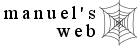convert inches & centimeters nursing calculators search site map

 enter length convert to inches convert to centimeters result

 solution

## Directions

Enter length in box to be converted.  Select either inches or centimeters button.  Press "convert" button to see result at bottom of calculator.

## Formula

cm  x  0.39* = in

in  x  2.54 = cm

## Examples

How tall is a woman in centimeters who is 5' 5" (65 in)?

5 foot  x 12 inches = 60 inches

60 inches + 5 inches = 65 inches

65 inches  x  2.54 = 165 centimeters

If a baby is 64 centimeters long, what is her length in inches?

64 centimeters  x  0.39 = 25 inches

*Shown as 0.39 after rounding to the nearest hundredth.  Program uses 0.3937008 to convert centimeters to inches